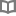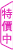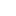中國酒文化：酒史×酒俗×敬酒辭×酒令，暢談杯中忘憂物，細數古今風流事 - 趙惠玲、羅烈文 - BOOKWALKER中文電子書

中國酒文化：酒史×酒俗×敬酒辭×酒令，暢談杯中忘憂物，細數古今風流事

趙惠玲、羅烈文

趙惠玲，七年級生，熱愛中華文化成痴，「民俗學」研究者，曾有親自接觸中國數個少數民族的經驗。因從小常聽父母提及中國傳統民間故事，對此興趣十分濃厚，一心決意編撰一本關於中華民俗傳統的書。著有《俗：文化，習俗，傳統，禁忌》、《令人理解不能的婚俗》。 羅烈文，專業編者。

• 放入購物車
•試閱

• 放入購物車
•試閱• 放入購物車
•試閱

• 放入購物車
•試閱

• 放入購物車
•無試閱

• 放入購物車
•試閱

• 放入購物車
•試閱

• 放入購物車
•試閱• 放入購物車
•試閱• 放入購物車
•試閱

• 放入購物車
•試閱

• 放入購物車
•試閱

• 放入購物車
•試閱

• 放入購物車
•試閱• 放入購物車
•試閱

• 放入購物車
•試閱

• 放入購物車
•試閱

• 放入購物車
•試閱

• 放入購物車
•試閱

• 放入購物車
•試閱

• 放入購物車
•試閱

• 放入購物車
•試閱

• 放入購物車
•試閱• 放入購物車
•試閱

• 放入購物車
•試閱

• 放入購物車
•試閱

• 放入購物車
•試閱

• 放入購物車
•試閱

• 放入購物車
•試閱

• 放入購物車
•試閱

• 放入購物車
•試閱

• 放入購物車
•試閱

• 放入購物車
•試閱

• 放入購物車
•試閱• 放入購物車
•無試閱

• 放入購物車
•試閱

• 放入購物車
•試閱

• 放入購物車
•試閱

• 放入購物車
•試閱

• 放入購物車
•試閱

• 放入購物車
•試閱

• 放入購物車
•試閱

• 放入購物車
•試閱

• 放入購物車
•試閱

• 放入購物車
•試閱

• 放入購物車
•試閱